# Liquid properties versus temperature

## 1. Introduction

Here is the process to define the liquid thermal characteristics from the importation of series of points representing the considered quantity curve listed in an Excel file. In the following example water mass density is considered, however, the same principle is applied for all other liquid thermal quantity which are defined below.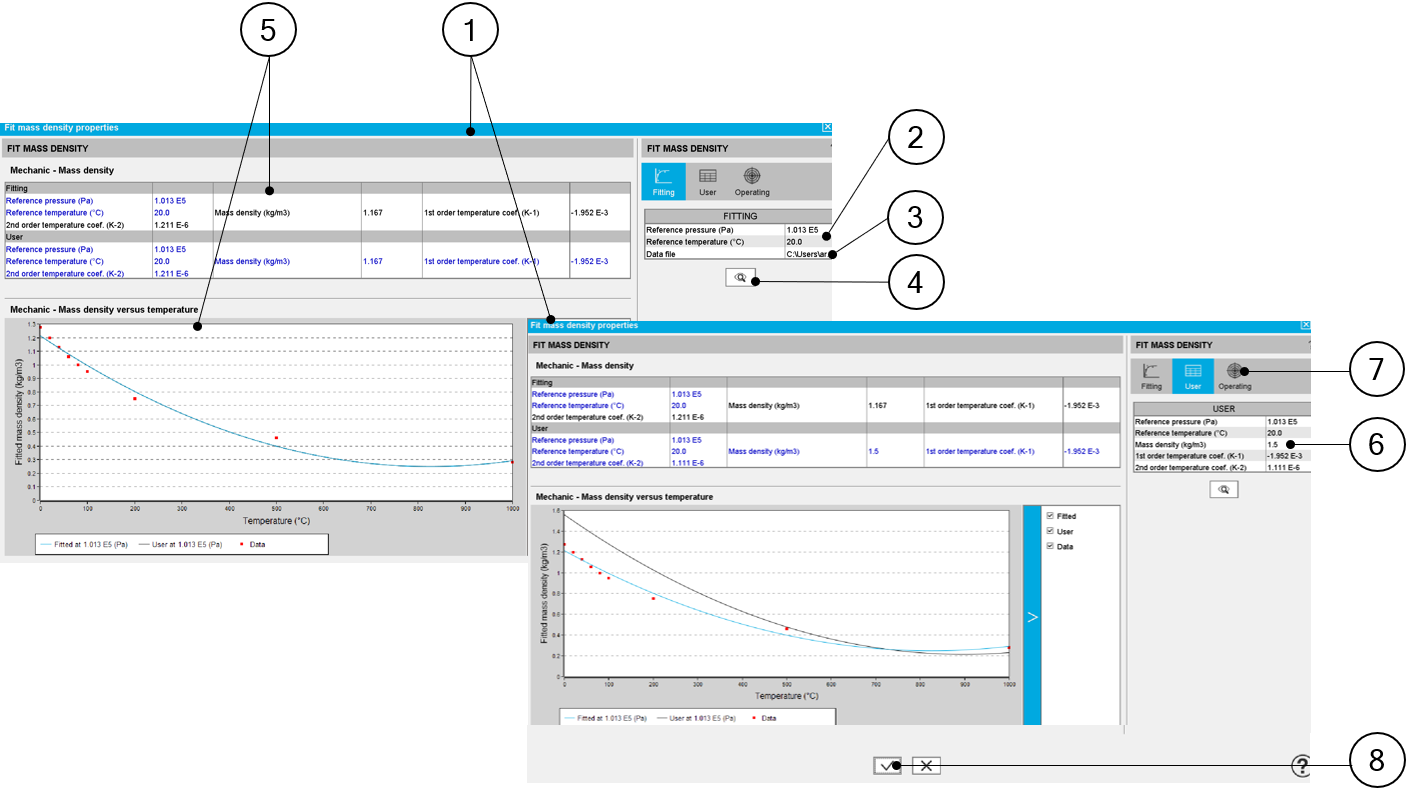Identification of the water mass density curve characteristics (for instance) 1 Dialog box allowing the characterization of the density curve imported from an Excel file 2 Select the reference temperature associated with the measures contained in the Excel file. 3 Path where Excel file containing the measures is stored. See an example of Excel file below. 4 Click on this button to import the Excel data. 5 When importing an Excel file, points representing the density curve are listed, an optimization process automatically computes and displays the corresponding characteristics. Three curves are displayed: Red points are the imported points (listed in the Excel file) Blue curve is the resulting curve computed by the optimization process. This corresponds to the computed characteristics and it is displayed just after the computation. The black curve shows a new curve generated when the parameters are changed by the user (see point 6) 6 Indeed, going to the tab “User” the user can adjust one or all the main parameters of the density curve. The density at reference temperature The density first order temperature coefficient The density second order temperature coefficient Reference temperature can also be adjusted in this tab. 7 Lastly parameter values, written in the input fields are validated when the user clicks on this button. It is possible to cancel the creation of the density curve model. In this case, the previous values defined before opening this dialog box are reset.

Example of an Excel file to define the water mass density curve parameters.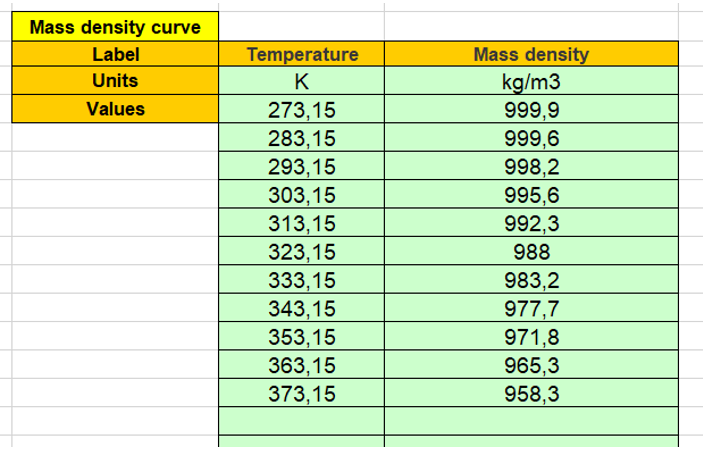Example of an Excel file to define the water mass density curve parameters

## 2. Mass density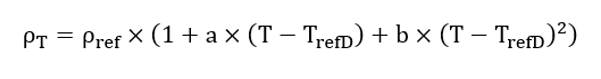Symbol Definition Unit T refD Mass density reference temperature T refD °C r T Mass density at T refD kg/m3 a Mass density first order temperature coefficient at T refD K-1 b Mass density second order temperature coefficient at T refD K-2
Note: Liquids are considered as incompressible in FluxMotor. Their properties are constant versus the pressure.

## 3. Dynamic viscosity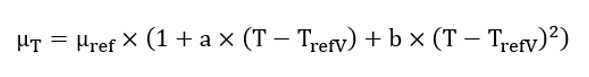Symbol Definition Unit T refV Dynamic viscosity reference temperature °C m ref Dynamic viscosity at T refV kg/m/s a Dynamic viscosity first order temperature coefficient at T refV K-1 b Dynamic viscosity second order temperature coefficient at T refV K-2

## 4. Thermal conductivity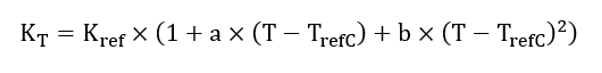Symbol Definition Unit T refC Thermal conductivity reference temperature °C K ref Thermal conductivity at T refC W/K/m a Thermal conductivity first order temperature coefficient at T refC K-1 b Thermal conductivity second order temperature coefficient at T refC K-2
Note: Liquids are considered as incompressible in FluxMotor. Their properties are constant versus the pressure.

## 5. Specific heat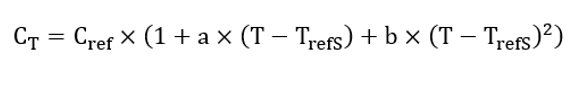Symbol Definition Unit T refS Specific heat reference temperature - T refS (°C) °C C ref Specific heat at T refS J/K/Kg a Specific heat first order temperature coefficient at T refS K-1 b Specific heat second order temperature coefficient at T refS K-2
Note: Liquids are considered as incompressible in FluxMotor®. Their properties are constant versus the pressure.

## 6. Thermal expansion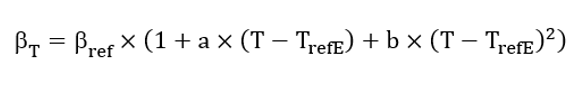Symbol Definition Unit T refE Thermal expansion reference temperature °C b ref Thermal expansion coefficient at T refE K-1 a Thermal expansion first order temperature coefficient at T refE K-1 b Thermal expansion second order temperature coefficient at T refE K-2